A bungee jumper of mass 75kg is attached to a bungee cord of length L=35m. She walks oﬀ a platform (with no initial speed), reaches a height

Question

A bungee jumper of mass 75kg is attached to a bungee cord of length L=35m. She walks oﬀ a platform (with no initial speed), reaches a height of 72m below the platform, and continues to oscillate. While air resistance eventually slows her to a stop, assume there is no air resistance for these calculations.

1. What is the spring constant of her bungee cord?
2. What is her speed when she is 35m below the platform (i.e., just before the cord starts to stretch)?
3. If she had instead jumped vertically oﬀ the platform, would the maximum displacement of the bungee cord increase, decrease, or stay the same?

in progress 0
5 months 2021-08-04T12:15:53+00:00 1 Answers 10 views 0

1. 77.31 N/m

2. 26.2 m/s

3. increase

Explanation:

1. According to the law of energy conservation, when she jumps from the bridge to the point of maximum stretch, her potential energy would be converted to elastics energy. Her kinetic energy at both of those points are 0 as speed at those points are 0.

Let g = 9.8 m/s2. And the point where the bungee ropes are stretched to maximum be ground 0 for potential energy. We have the following energy conservation equation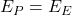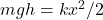where m = 75 kg is the mass of the jumper, h = 72 m is the vertical height from the jumping point to the lowest point, k (N/m) is the spring constant and x = 72 – 35 = 37 m is the length that the cord is stretched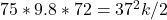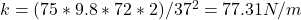2. At 35 m below the platform, the cord isn’t stretched, so there isn’t any elastics energy, only potential energy converted to kinetics energy. This time let’s use the 35m point as ground 0 for potential energy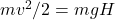where H = 35m this time due to the height difference between the jumping point and the point 35m below the platform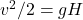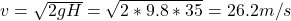3. If she jumps from her platform with a velocity, then her starting kinetic energy is no longer 0. The energy conservation equation would then be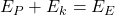So the elastics energy would increase, which would lengthen the maximum displacement of the cord